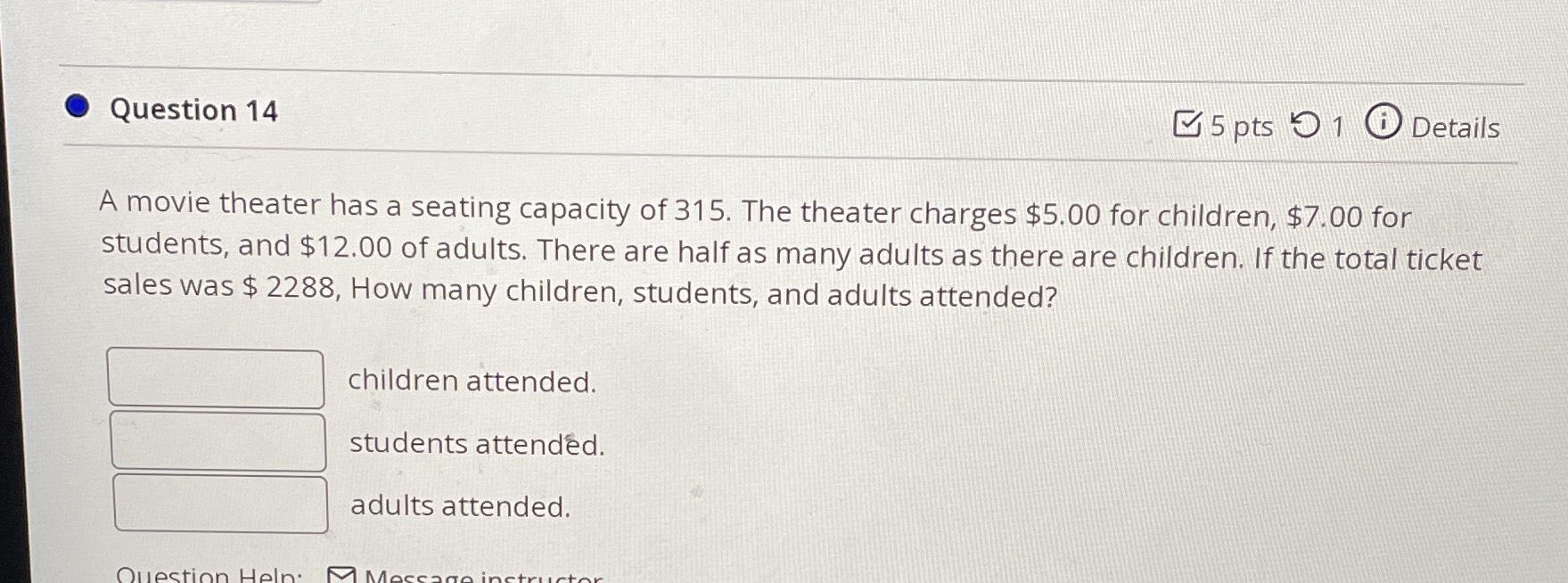### ¿Todavía tienes preguntas de matemáticas?

Pregunte a nuestros tutores expertos
Algebra
PreguntaA movie theater has a seating capacity of $$315$$ . The theater charges $$\ 5.00$$ for children, $$\ 7.00$$ for students, and $$\ 12.00$$ of adults. There are half as many adults as there are children. If the total ticket sales was $$\ 2288 ,$$ How many children, students, and adults attended?